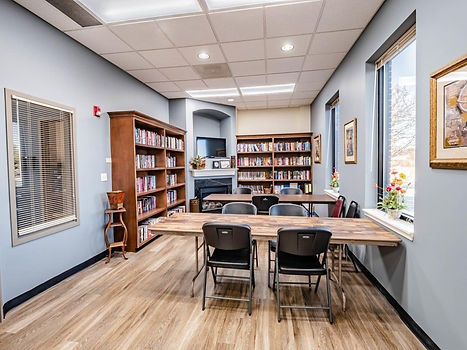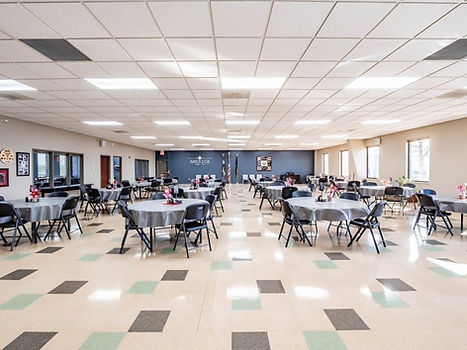top of page

Commercial/Private Hall (M-F)

Deposit=\$250

Rental=\$250

Non-Profit (M-F)

Deposit=\$200

Rental=\$175Commercial/Private Activity Room (M-F)

Deposit=\$100

Rental=\$100

Non-Profit (M-F)

Deposit=\$30

Rental=\$30Commercial/Private Library (M-F)

Deposit=\$60

Rental=\$60

Non-Profit (M-F)

Deposit=\$25

Rental=\$25Commercial/Private Hall (S-S)(Holidays)

Deposit=\$500

Rental=\$625

Non-Profit (S-S)

Deposit=\$150

Rental=\$250

Commercial/Private Library (S-S)

Deposit=\$60

Rental=\$60

Non-Profit (S-S)

Deposit=\$40

Rental=\$40Commercial/Private Activity Room (S-S)

Deposit=\$100

Rental=\$200

Non-Profit (M-F)

Deposit=\$50

Rental=\$150The use of the kitchen is an additional \$200.00